# pp_notebook

## Postprocessing with atooms¶

In this notebook, we are going to show how to perform standard analysis of molecular dynamics trajectories using the atooms package and its postprocessing library. You can install both of them from pypi.

In :
%matplotlib inline
import pylab as pl
import sys


We then import the postprocessing library and the trajectory class from atooms. In this particular case, we will read an XYZ file, so we load the TrajectoryXYZ class

In :
import atooms.postprocessing as pp
from atooms.trajectory import TrajectoryXYZ


We consider an example trajectory, located in the postprocessing package. We can grab it at this link https://gitlab.info-ufr.univ-montp2.fr/atooms/postprocessing/raw/master/data/kalj-small.xyz (we can download it from a terminal with wget). Let's store the path to this file in a variable for later convenience

In :
path = "kalj-small.xyz"


### Handling a trajectory¶

A trajectory is an object with many properites. To load a trajectory, we create an instance of the class as follows

In :
th = TrajectoryXYZ(path)


The trajectory is a list-like object, in the sense that it can be iterated up and sliced. Each frame of the trajectory contains a System object, which is a full configration of the system at a given instant of time during the simulation. Here we print the first, the 10th and the last frame

In :
print th, th, th[-1]

<atooms.system.system.System object at 0x7f769d54be90> <atooms.system.system.System object at 0x7f769d54be10> <atooms.system.system.System object at 0x7f769d54bdd0>


To know how many frames we have

In :
print len(th)

101


To clarify: a slice is a list of frames, not a trajectory

In :
th[10: 15]

Out:
[<atooms.system.system.System at 0x7f769d6a2390>,
<atooms.system.system.System at 0x7f769d6b9a10>,
<atooms.system.system.System at 0x7f769d6520d0>,
<atooms.system.system.System at 0x7f769d662750>,
<atooms.system.system.System at 0x7f769d672dd0>]

Note: it is actually possible to define a slice of a trajectory as a trajectory by using the Sliced class decorator, see below.

If the trajectory contains metadata, these can be retrieved directly:

In :
print "Timestep during the trajectory:", th.timestep
print "Steps corresponding to the 3rd frame:", th.steps

Timestep during the trajectory: 0.001
Steps corresponding to the 3rd frame: 2000
{'ndim': 3, 'npart': 150, 'cell': [5.0, 5.0, 5.0], 'step': 0, 'dt': 0.001, 'columns': ['name', 'pos']}


### Analysis of the trajectory¶

Now that we have constructed a trajectory object, we can proceed to the analysis. We are going to consider two main aspects of the analysis:

• structural correlations
• dynamical correlations

#### Structural correlations : computing the radial distribution function¶

The radial distribution function g(r) describes how density varies as a function of the radial distance from a reference particle. In liquids, one normally averages over all particles, obtaining a descriptor of the probability to find a second particle a distance $r$ from a given particle, relative to that of the ideal gas.

For $N$ particles of the same type at density $\rho$ it is

$$g(r)=\frac{1}{N\rho}\left\langle\sum_i^{N}\sum_{i\neq j}\delta(r-|\mathbf{r}_i-\mathbf{r}_j|)\right\rangle$$

.

Notice that the average number of particles with a distance $R$, i.e. the average coordination $n(R)$, can be computed from the radial distribution function via integration in spherical coordinates (for 3D systems)

$$n(R)=4\pi \rho\int_0^R g(r)r^2 dr$$

In postprocessing the radial distribution function is a correlation object that acts on a trajectory. In order to compute it, we simpy construct the object, specifying some parameters, and then run the calculation with the do() method

In :
gr = pp.RadialDistributionFunction(th, norigins=5, dr=0.04)
gr.do()


Once the calculation is performed, the radial distribution object gr contains (like all correlators in postprocessing) two arrays:

• the grid array contains the independent variable (or variables), binned according to our input parameters (in this case, the smallest space interval that we resolve, dr
• the value array contains the actual value of teh computation, in this case the values of the g(r)

We can directly plot the results with

In :
pl.plot(gr.grid, gr.value)
pl.xlabel("r"), pl.ylabel("g(r)");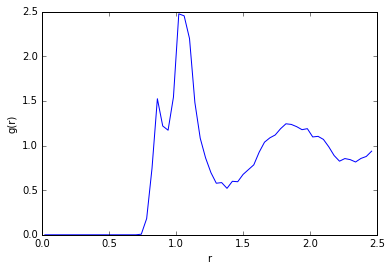As we can see, the function displays two narrow peaks around $r=1$ and a broader peak further away. The several peaks correspond to the fact that the trajectory actualy contains two types of particles, noted as "A" and "B".

We can compute separate distribution functions for the A and B particles and also the cross distribution funtion for the probability to find a particle B at distance $r$ from particel $A$ using the Partial class in postprocessing:

In :
gr = pp.Partial(pp.RadialDistributionFunction, species=['A', 'B'], trajectory=th, norigins=100)
gr.do()


In this case, the result contains a dictionary gr.partial:

In :
type(gr.partial)

Out:
dict

We can treat the result as any norrmal dictionary:

In :
for key,g in gr.partial.items():
pl.plot(g.grid, g.value, label=str("".join(key)))
pl.legend(); pl.xlabel("r"); pl.ylabel(r"$g_{\alpha\beta}(r)$");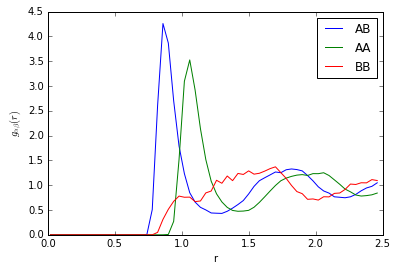Sometimes, it is useful to analyse only sections of a trajectory. To this purpose, one can slice the trajectory using atooms and analyse individual frames or subsets of frames.

In :
from atooms import trajectory

t =  trajectory.Sliced(th, slice(-1, len(th))) # analyse only the last frame
gr.do()
pl.plot(gr.grid, gr.value)
pl.xlabel("r"); pl.ylabel("g(r)"); # notice that the g(r) is more noisy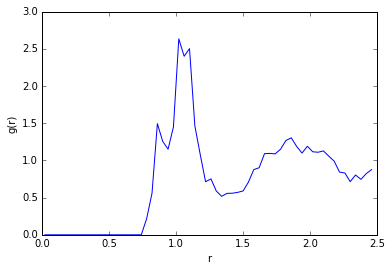#### Dynamical Correlations: computing the Mean Square Displacement¶

A very similar kind of anaysis can be performed on dynamical quantities, which quantify correlations in time.

The most elementary of such quantities is the mean squared displacement (MSD). This is defined as

$$MSD(t)= \langle \mathbf{r}(t-t_0) - \mathbf{r}(t_0)\rangle$$

The average is normally perfomed over all the paricles $N$ and over multiple values for the origin of time $t_0$.

The analysis process is now familiar. First we cosntruct the msd object and then perform the calculation with .do.

In :
msd = pp.MeanSquareDisplacement(th)
msd.do()

In :
pl.loglog(msd.grid, msd.value, 'o')
pl.xlabel("t");  pl.ylabel("MSD(t)");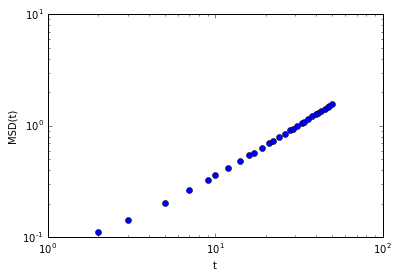Again, we can compute partial mean square displacements using the Partial class

In :
msds = pp.Partial(pp.MeanSquareDisplacement, species=['A','B'], trajectory=th, norigins=100)
msds.do()
pl.loglog(msds.partial['A'].grid, msds.partial['A'].value, 'o')
pl.loglog(msds.partial['B'].grid, msds.partial['B'].value, 'o')
pl.xlabel("t"); pl.ylabel("MSD(t)");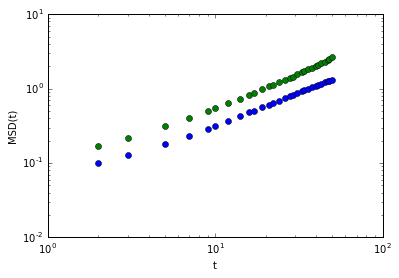### Computing the Self Intermediate Scattering Function¶

We compute the intermediate scattering function (ISF) at specific wave-vectors using a logarithmic time grid.

In :
isf = pp.Partial(pp.SelfIntermediateScattering, species=["A"], trajectory= th, kgrid=[2*pl.pi, 2.5*pl.pi], nk=1, tgrid=pl.logspace(0, 3, base=10))
isf.do()


To get some info on the parameters passed to compute the ISF, have a look at the help for the base class

In :
help(pp.fourierspace.FourierSpaceCorrelation)

Help on class FourierSpaceCorrelation in module atooms.postprocessing.fourierspace:

class FourierSpaceCorrelation(atooms.postprocessing.correlation.Correlation)
|  Base class for Fourier space correlation functions.
|
|  The correlation function is computed for each of the scalar values
|  k_i of the provided kgrid. If the latter is None, the grid is
|  built using ksamples entries linearly spaced between kmin and
|  kmax.
|
|  For each sample k_i in kgrid, the correlation function is
|  computed over at most nk wave-vectors (k_x, k_y, k_z) such that
|  their norm (k_x^2+k_y^2+k_z^2)^{1/2} lies within dk of the
|  prescribed value k_i.
|
|  Method resolution order:
|      FourierSpaceCorrelation
|      atooms.postprocessing.correlation.Correlation
|      __builtin__.object
|
|  Methods defined here:
|
|  __init__(self, trajectory, grid, symbol, short_name, description, phasespace, nk=8, dk=0.1, kmin=-1, kmax=10, ksamples=20)
|
|  report(self, k_sorted, k_selected)
|
|  ----------------------------------------------------------------------
|  Methods inherited from atooms.postprocessing.correlation.Correlation:
|
|  __call__(self)
|
|  __str__(self)
|
|      Add filter callback cbk along with positional and keyword arguments
|
|  analyze(self)
|      Subclasses may implement this and store the results in the self.results dictonary
|
|  compute(self)
|      Compute the correlation function.
|
|      It wraps the _compute() method implemented by subclasses.
|      This method also returns the tuple self.grid, self.value.
|
|  do(self)
|      Do the full template pattern: compute, analyze and write the
|      correlation function.
|
|      Read correlation function from existing file
|
|  write(self)
|      Write the correlation function and the analysis data to file
|
|  ----------------------------------------------------------------------
|  Data descriptors inherited from atooms.postprocessing.correlation.Correlation:
|
|  __dict__
|      dictionary for instance variables (if defined)
|
|  __weakref__
|      list of weak references to the object (if defined)
|
|  ----------------------------------------------------------------------
|  Data and other attributes inherited from atooms.postprocessing.correlation.Correlation:
|
|  nbodies = 1



The ISF decays to zero at long times, as it should in an ergodic liquid.

In :
pl.semilogx(isf.partial['A'].grid, isf.partial['A'].value, '-o')

Out:
[<matplotlib.lines.Line2D at 0x7f769d0da4d0>]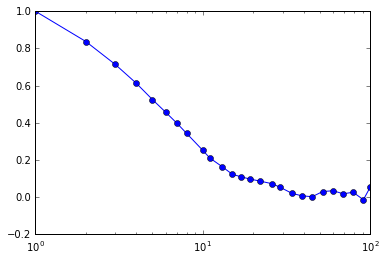We can extend the correlation class to compute additional correlation functions. The particle coordinates are loaded into the _pos instance variable as a list of numpy arrays. Each numpy array is a (ndim, npart) representation of the full particles' coordinates in a given frame of the trajectory. This is a gist of a new correlation class.

In :
from collections import defaultdict
import numpy

def some_function(x, pos):
return 0.0

class NewCorrelation(pp.correlation.Correlation):
def __init__(self, trajectory, grid):
pp.correlation.Correlation.__init__(self, trajectory, grid, phasespace=["pos"])

def _compute(self):
print "Computing new correlation"
raw = defaultdict(list)
for i in range(len(self._pos)):
for x in self.grid:
raw[x].append(some_function(x, self._pos[i]))
self.value = [numpy.mean(raw[x]) for x in raw]

In :
nw = NewCorrelation(th, [0.0, 1.0])
nw.compute()

Computing new correlation

Out:
([0.0, 1.0], [0.0, 0.0])

Author: Francesco Turci

In [ ]: